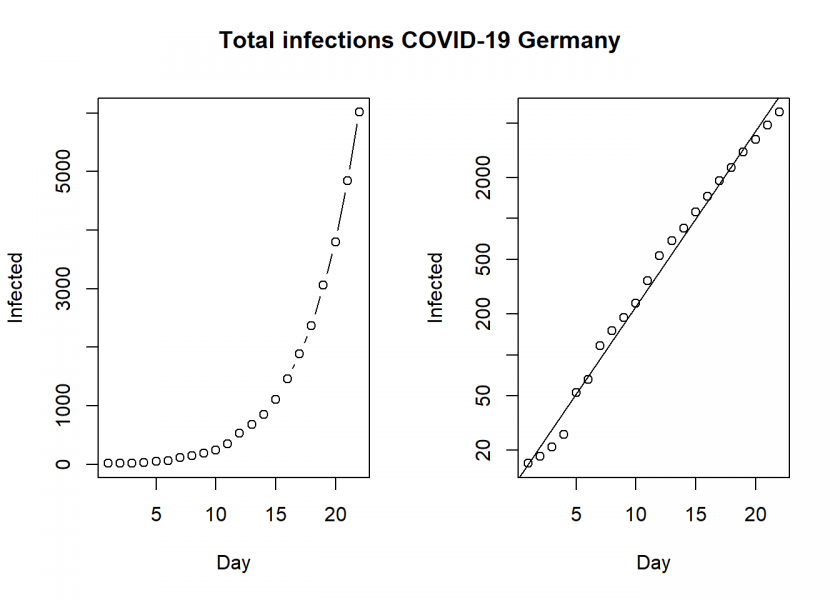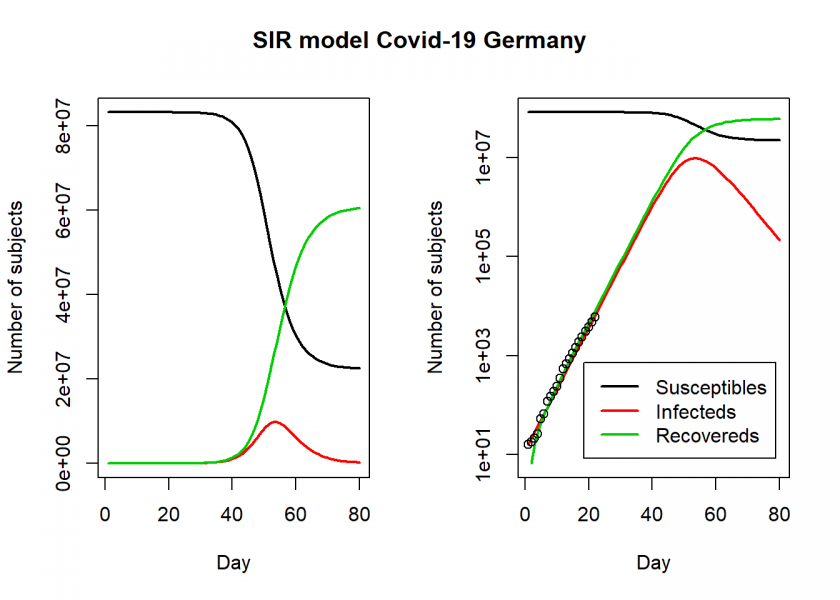Want to share your content on R-bloggers? click here if you have a blog, or here if you don't.It is such a beautiful day outside, lot’s of sunshine, spring at last… and we are now basically all grounded and sitting here, waiting to get sick.

So, why not a post from the new epicentre of the global COVID-19 pandemic, Central Europe, more exactly where I live: Germany?! Indeed, if you want to find out what the numbers tell us how things might develop here, read on!

We will use the same model we already used in this post: Epidemiology: How contagious is Novel Coronavirus (2019-nCoV)?. You can find all the details there and in the comments.

```library(deSolve)

# https://en.wikipedia.org/wiki/2020_coronavirus_pandemic_in_Germany#Statistics
Infected <- c(16, 18, 21, 26, 53, 66, 117, 150, 188, 240, 349, 534, 684, 847, 1112, 1460, 1884, 2369, 3062, 3795, 4838, 6012)
Day <- 1:(length(Infected))
N <- 83149300 # population of Germany acc. to Destatis

old <- par(mfrow = c(1, 2))
plot(Day, Infected, type ="b")
plot(Day, Infected, log = "y")
abline(lm(log10(Infected) ~ Day))
title("Total infections COVID-19 Germany", outer = TRUE, line = -2)
```This clearly shows that we have an exponential development here, unfortunately as expected.

```SIR <- function(time, state, parameters) {
par <- as.list(c(state, parameters))
with(par, {
dS <- -beta/N * I * S
dI <- beta/N * I * S - gamma * I
dR <- gamma * I
list(c(dS, dI, dR))
})
}

init <- c(S = N-Infected, I = Infected, R = 0)
names(parameters) <- c("beta", "gamma")
out <- ode(y = init, times = Day, func = SIR, parms = parameters)
fit <- out[ , 3]
sum((Infected - fit)^2)
}

Opt <- optim(c(0.5, 0.5), RSS, method = "L-BFGS-B", lower = c(0, 0), upper = c(1, 1)) # optimize with some sensible conditions
Opt\$message
##  "CONVERGENCE: REL_REDUCTION_OF_F <= FACTR*EPSMCH"

Opt_par <- setNames(Opt\$par, c("beta", "gamma"))
Opt_par
##      beta     gamma
## 0.6428120 0.3571881

t <- 1:80 # time in days
fit <- data.frame(ode(y = init, times = t, func = SIR, parms = Opt_par))
col <- 1:3 # colour

matplot(fit\$time, fit[ , 2:4], type = "l", xlab = "Day", ylab = "Number of subjects", lwd = 2, lty = 1, col = col)
matplot(fit\$time, fit[ , 2:4], type = "l", xlab = "Day", ylab = "Number of subjects", lwd = 2, lty = 1, col = col, log = "y")
## Warning in xy.coords(x, y, xlabel, ylabel, log = log): 1 y value <= 0
## omitted from logarithmic plot

points(Day, Infected)
legend("bottomright", c("Susceptibles", "Infecteds", "Recovereds"), lty = 1, lwd = 2, col = col, inset = 0.05)
title("SIR model Covid-19 Germany", outer = TRUE, line = -2)
``````par(old)

R0 <- setNames(Opt_par["beta"] / Opt_par["gamma"], "R0")
R0
##       R0
## 1.799646

fit[fit\$I == max(fit\$I), "I", drop = FALSE] # height of pandemic
##          I
## 54 9769398

max_infected <- max(fit\$I)
max_infected / 5 # severe cases
##  1953880

max_infected * 0.06 # cases with need for intensive care
##  586163.9

# https://www.newscientist.com/article/mg24532733-700-why-is-it-so-hard-to-calculate-how-many-people-will-die-from-covid-19/
max_infected * 0.007 # deaths with supposed 0.7% fatality rate
##  68385.78
```

So, according to this model, the height of the pandemic will be reached by the end of April, beginning of May. About 10 million people would be infected by then, which translates to about 2 million severe cases, about 600,000 cases in need of intensive care and up to 70,000 deaths.

Those are the numbers our model produces and nobody knows whether they are correct while everybody hopes they are not. One thing has to be kept in mind though: the numbers used in the model are from before the shutdown (for details see here: DER SPIEGEL: Germany Moves To Shut Down Most of Public Life). So hopefully those measures will prove effective and the actual numbers will turn out to be much, much lower.

I wish you all the best and stay healthy!